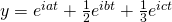## Wheels on Wheels on Wheels

July 4th, 2009 | Categories: general math, mathematica, Wolfram Demonstrations | Tags:

Take a wheel of radius 1 and set it rotating about its axis with a frequency of 1 turn per second. Attach a second wheel, of radius 1/2, to the circumference of the first and set this second wheel rotating about its axis at a frequency of 7 turns per second. Finally, attach a third wheel to the circumference of the second and set this wheel to rotate about it’s axis at a frequency of 17 turns per second.

Now, consider a point on the circumference of the third wheel. What pattern will it trace out as the three wheels rotate?  Click on the video below to find out.

I first came across this idea in a Wolfram demonstration by Daniel de Souza Carvalho.  Daniel’s demonstration focused on the fact that you could write down the equations of these curves in two different ways. If the wheels are rotating with frequencies a, b and c respectively then you can either describe the corresponding curve with a pair of parametric equations as follows:or as a complex valued equation:This was a nice demonstration but I wanted to see what sort of patterns I could get by changing the frequencies of the wheels. So, I downloaded Daniel’s demonstration, added some sliders and tick boxes and then uploaded the result. Wolfram Research cleaned up my code a bit and the result was published as the Wolfram Demonstration Wheels on Wheels on Wheels.

It turns out that you can get a LOT of different patterns out of this system as you can see below.These systems were considered in the paper “Wheels on Wheels on Wheels—Surprising Symmetry,” Mathematics Magazine 69(3), 1996 pp. 185–189 by F. A. Farris. In this paper, Farris showed that the resulting curve exhibits m-fold symmetry if the three frequencies are congruent (mod m).

Can you think of any interesting variations to this system?

Update (6th July 2009): Taki has written another version of this demonstration which includes an animation of the wheels and also looked at an example with four wheels over at his blog, Mesh Mess.

1. Just an amateurs guess, but if you vary the size of the circles you should get similar symmetry patterns up to a point and then they would change to something else. Would that new pattern have the same symmetries? I don’t know.
This is too easy to be new through. For an attempt at something more original, what about varying the radius of the circles with some frequency?

2. Wasn’t there a simple version of this sold many years back- where you had a wheel and many different “inner wheels” that drew different shapes?

You also used different colour pens?

3. The plastic version sold in stores was called Spirograph. I’ve been looking to buy it at a second hand store, but no luck so far. I had one when I was a kid, and I enjoyed it.

Check out an online Spirograph at http://learninginmathland.blogspot.com/2009/05/spirograph-math.html

4. There is a Wolfram Demonstration for Spirographs too

http://demonstrations.wolfram.com/Spirograph/

The mathematics is very similar to the wheels on wheels on wheels system.

5. @Weldon MacDonald
but the 1st step is to make a model of three wheels revolving with different frequencies…
how will you make it?